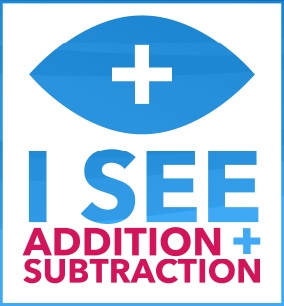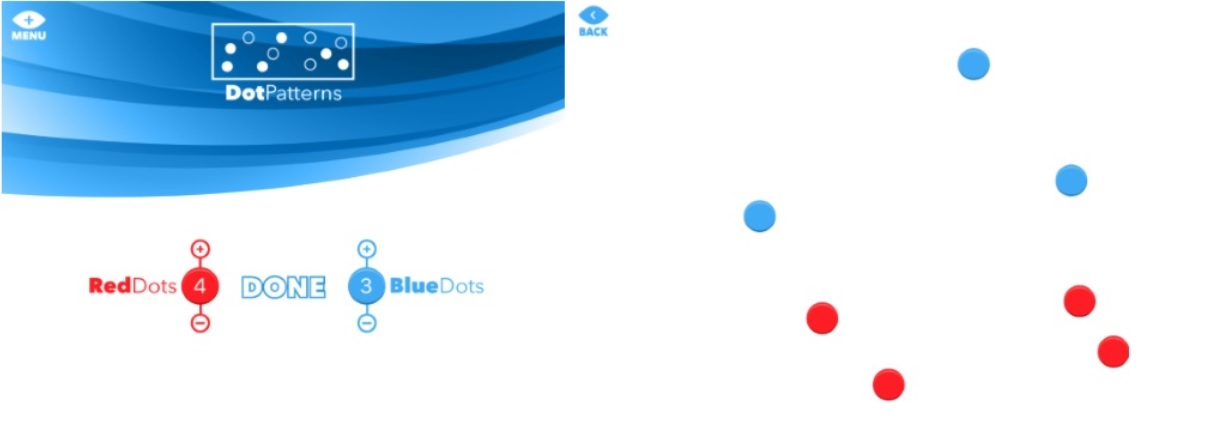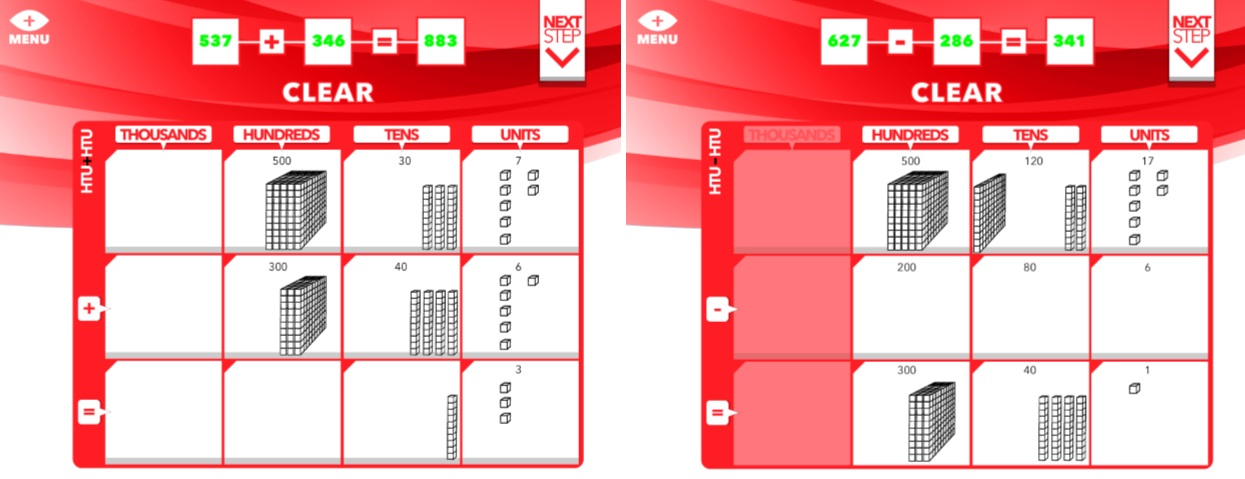# I See Addition and SubtractionAddition and subtraction calculations are made visual by I See Maths + -. This iPad app allows educators to create a range of images that help children to understand key calculation concepts.

The Singapore Bar feature allows the 'part-part-whole' structure to be made visible; calculations can be shown on 10-frames and the 100-square; column + and - can be modelled step-by-step. The images can also be adapted to create meaningful questions. This makes I See Maths + - an invaluable teaching tool.The 'part-part-whole' structure, showing the relationship between addition and subtraction, is modelled by the Singapore Bar. Once the calculation has been entered, different numbers can be hidden to create effective questions.Calculations within 30 can be modelled on 10-frames. This helps children to develop a 'feel' for the size of number and see how additions are broken down over the tens boundary.Calculations can be modelled on the 100-square, helping children to visualise number patterns when adding or subtracting 10 and 1. Challenge can be added by hiding any of the numbers on the 100-square.Dot pattern games, creating using one or two colours of dots, can be used to develop immediate recognition of small quantities. Equally, children learn to progress from a 'counting in ones' strategy when quickly recognising larger quantities.Column method additions and subtractions are modelled step-by-step using one, ten and hundred blocks. This helps children to develop a conceptual understanding of the processes being taught.